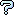All about flooble | fun stuff | Get a free chatterbox | Free JavaScript | Avatarsperplexus dot infoRising water levels (Posted on 2006-06-26)You have rectangular pot of water 10cm by 10cm at the bottom and 6 cm deep. It is filled to a depth of 3cm.

You have 7 solid steel shapes in front of you. The question is to find the new level of the water after each shape is put in the pot in the orientation described. The previous shape is removed before adding the next.

1. A cube 5cm on a side.

2. A prism in the shape of a right triangle with legs 5cm long. It is 5cm high but is placed on one of its legs with its hypotenuse sloping out of the water.

3. Another prism, this one having an equilateral triangle of sides 5cm and height 5cm. It is to be placed on its side with two faces sloping up out of the water.

4. A regular hexagonal prism. Each edge of the hexagon is 4cm and the height is 5cm. It is to be placed on its side.

5. A right square pyramid. Its base is 6cm on a side. It is 5cm high. It is to be placed base down.

6. A right cylinder of radius 3cm and length 5cm. It is to be placed on its side.

7a. A right cone of radius 3cm and height 5cm. It is to be placed base down.

7b. The same cone as 7a. This time placed on its side.

 No Solution Yet Submitted by Jer Rating: 4.1667 (6 votes)Comments: ( Back to comment list | You must be logged in to post comments.)re: #7b Solution (with explanation) -- a numerical integration comparison| Comment 22 of 26 |(In reply to #7b Solution (with explanation) by Dej Mar)

Not being familiar with the volume of wedge formula given by Dej Mar, I wanted to verify if a numerical integration agrees with what is given in his solution.

I am assuming the cone lying on its side has one whole slant height, from apex of the cone to one point on the base, lying on the bottom of the pot.

From the side, the cross section looks like an isosceles triangle with a 6-cm base slanting upward and a 5-cm altitude slanting at a gentler angle, meeting that base in the middle. The slant height, horizontally along the bottom, is sqrt(3^2+5^2)=5.830951894845301.

The volume of the whole cone is pi * 3^2 * 5/3 = 47.12388980384689.

The angle that the axis of the cone makes with the bottom of the pot is atan(3/5); call the measure of this angle A. The angle that the opposing slant height makes with the bottom is 2A.

The top of the circular base is 6 cos A above the bottom of the pot and 3 cos A above the level of the center of the circular base.

If in fact the water level is now 3.3823 cm above the bottom of the pot, it is 3.3823 - 3 cos A above the level of the center of the circular base.

We need to integrate the areas of the horizontal parabolic segments above the water level so we can subtract from the total volume of the cone. If we measure a y coordinate with zero at the level of the center of the circular base, then the integration is from y = 3.3823 - 3 cos A to 3 cos A.

The area of each parabolic segment is b * h * 2/3 where b is the length of the base and h is the distance from the base to the apex.

The circle can be taken as x^2 + y'^2 = 9, where y' = y / cos A, as y is measured vertically, but y' is along the plane of the circular base, tilted at angle A. The base of each parabolic segment is twice the x value found this way.

The height of the parabolic segment is in two parts, separated by a vertical line from the apex of the circular base.  On one side (toward the base) the length is (3*cosA-y)*tanA and on the other (in the direction of the apex of the cone), it's (3*cosA-y)/tan(2A).

Again, the area is 2/3 the base times the height, and the increment of volume is that area times the thickness of the lamina being added.

The following program does the numerical integration:

DEFDBL A-Z
pi = ATN(1) * 4

CLS
vWhole = pi * 9 * 5 / 3: PRINT vWhole

angle = ATN(3 / 5): PRINT angle * 180 / pi
angle2 = 2 * angle
apex = 3 * COS(angle): PRINT apex

d = 3.3823 - 3 * COS(angle): PRINT d

' y measured vertically from fattest part of circle

stp = .00001
FOR y = d TO apex STEP stp
y1 = y / COS(angle)
x = SQR(9 - y1 * y1)
ht = (apex - y) * TAN(angle) + (apex - y) / TAN(angle2)
area = 2 * x * ht * 2 / 3
vol = vol + area * stp
NEXT y

PRINT vWhole - vol

PRINT .3823 * 100

END

This results in the volume below the water level as 41.39853039775774, which should have added .414 to the water level, rather than .382. Also, as the interval of integration gets finer, the total seems to go up rather than down, getting farther from agreement with Dej Mar's solution.

 Posted by Charlie on 2006-07-03 14:23:21Please log in:

 Search: Search body:
Forums (0)